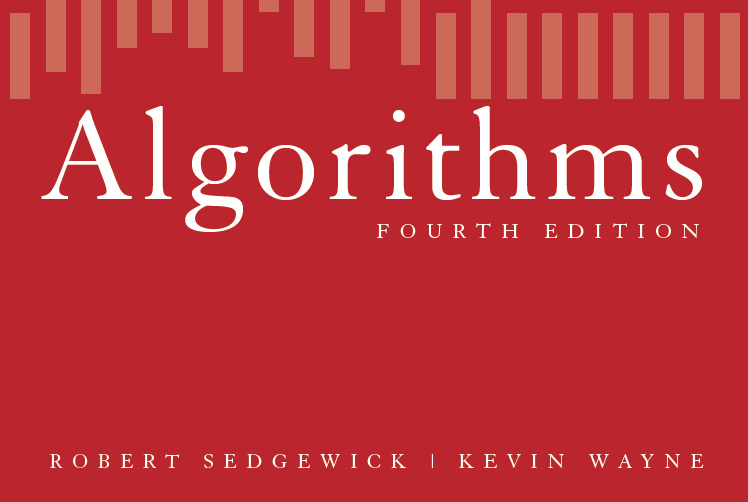【Princeton】算法(3)：排序

初级排序算法

排序规则

• 自反性（reflexive）：$v = v$
• 反对称性（antisymmetry）：$v \le w$且$w \le v$，则$v = w$
• 传递性（transitivity）：$v \le w$且$w \le x$，则$v \le x$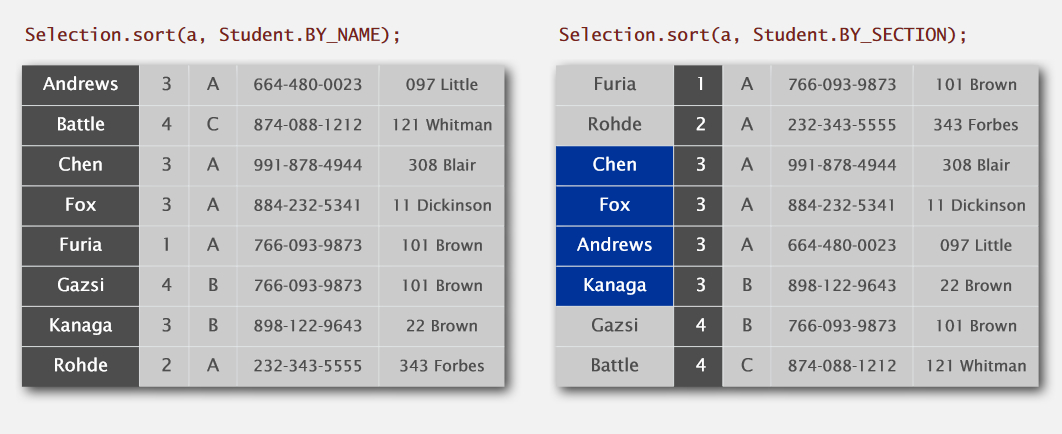选择排序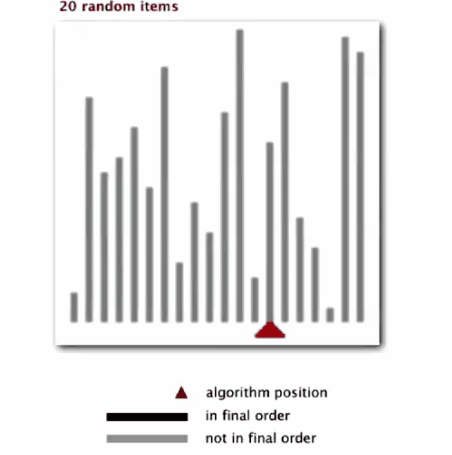插入排序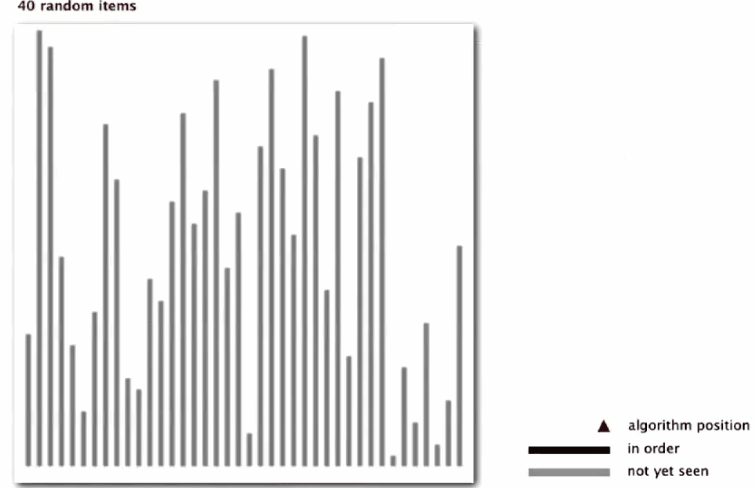• 数组中各元素距其最终位置都不太远
• 一个有序的大数组后接一个小数组
• 数组中只有个别元素位置不对

希尔排序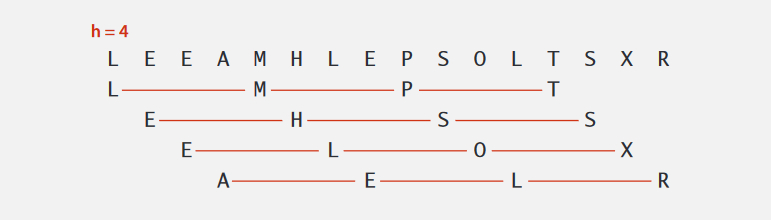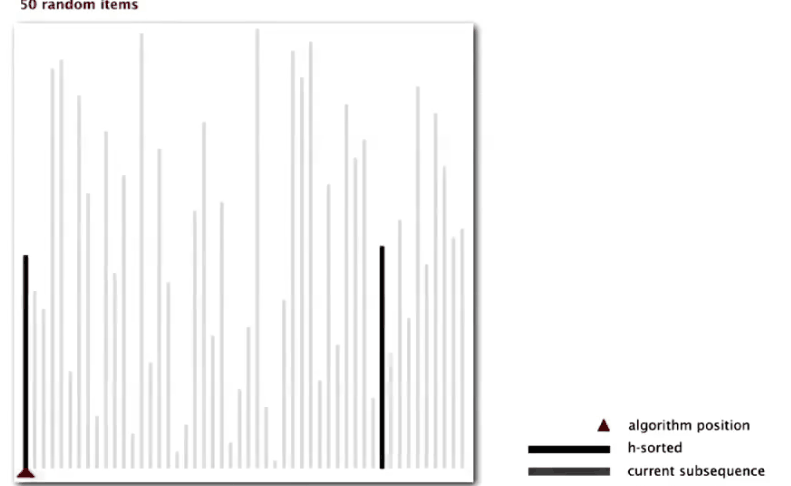归并排序

实现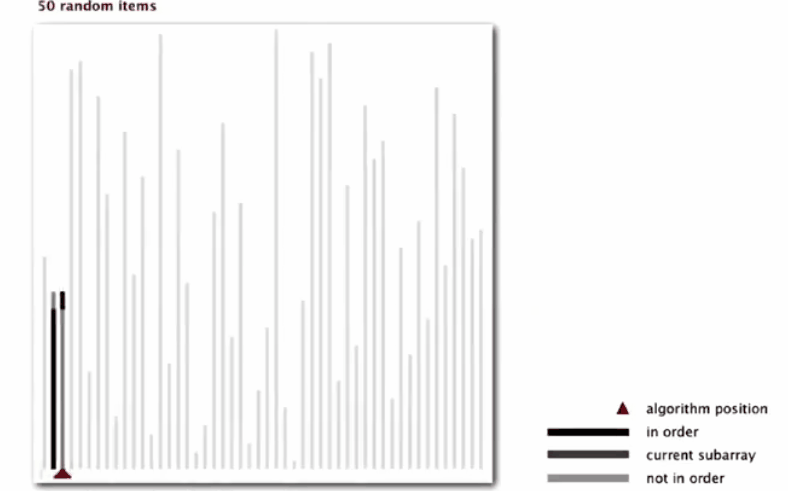证明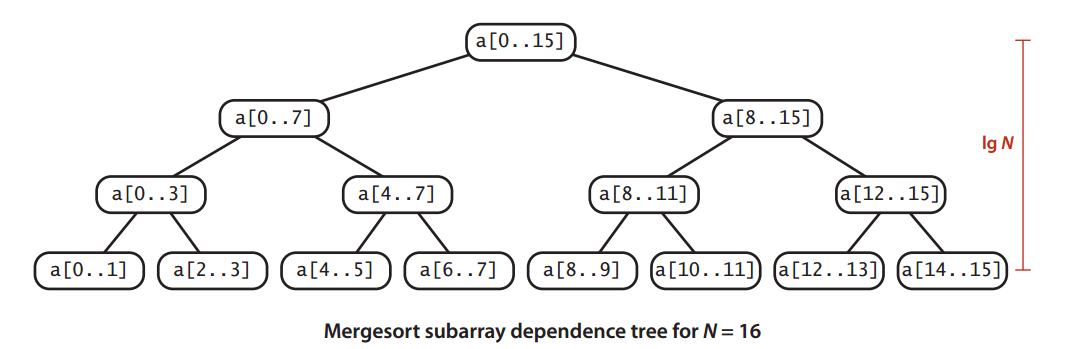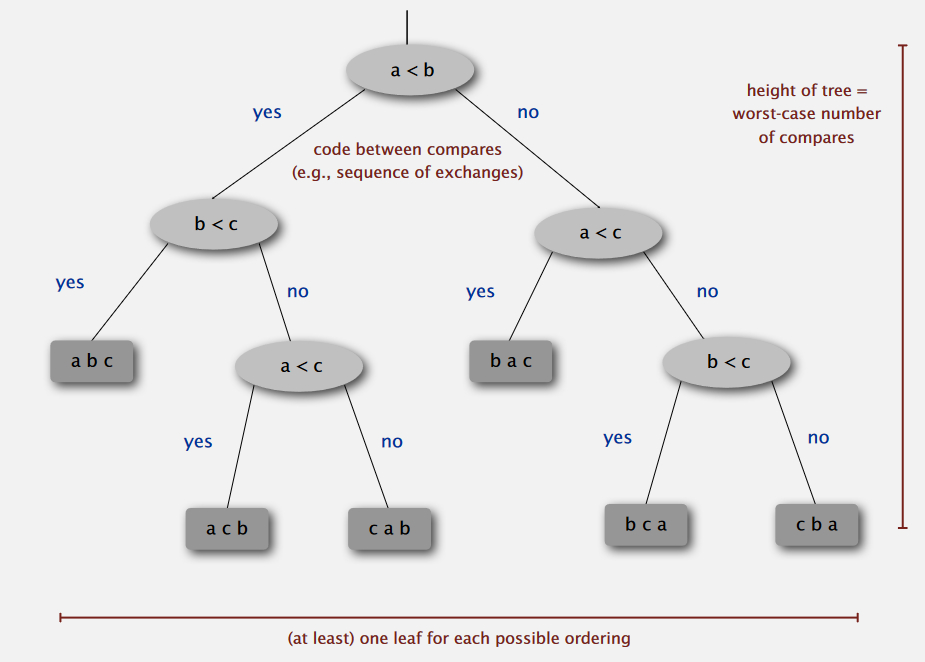• 数组中元素初始时已有一定顺序，排序时将不需要进行$N\log N$次比较
• 数组中元素中包含重复项，排序也不需要进行$N\log N$次比较

改进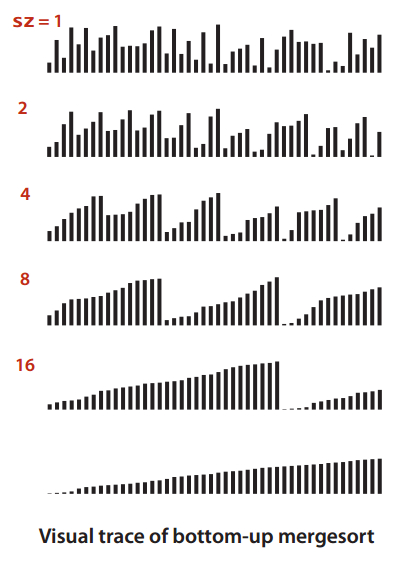快速排序

实现

• a[lo]到a[j-1]中所有的元素都不大于a[j]
• a[j+1]到a[hi]中所有的元素都不小于a[j]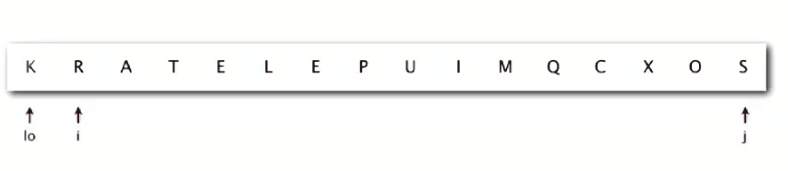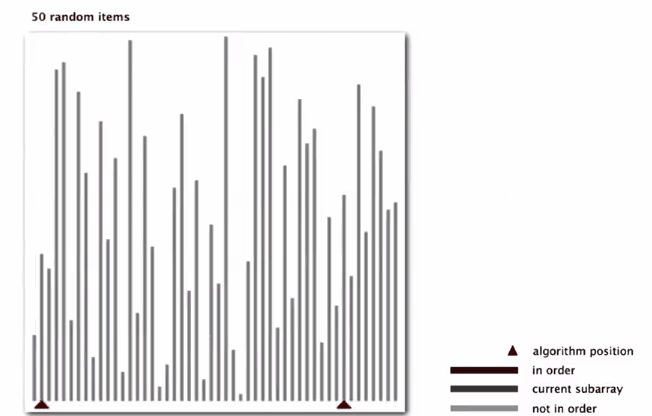改进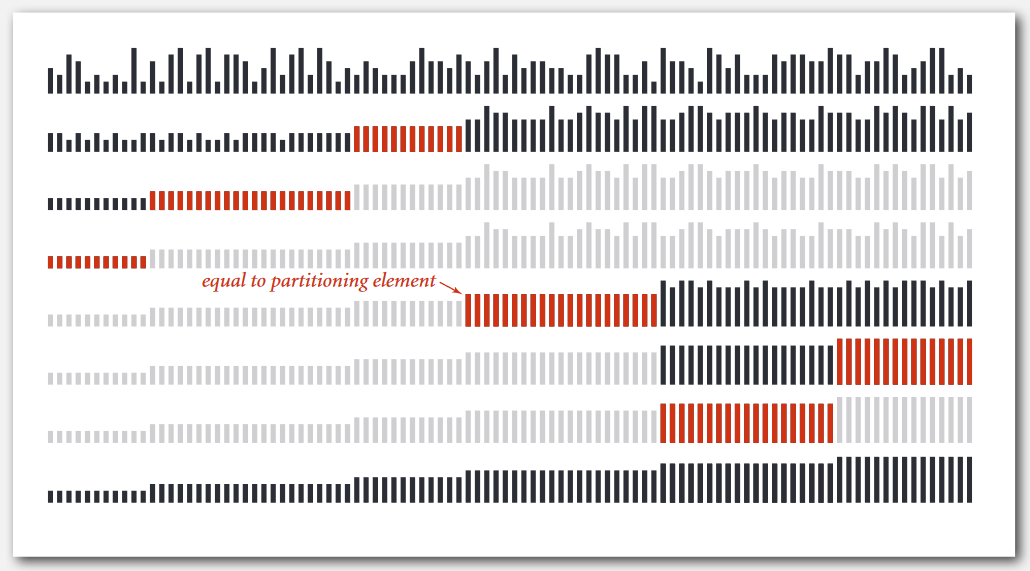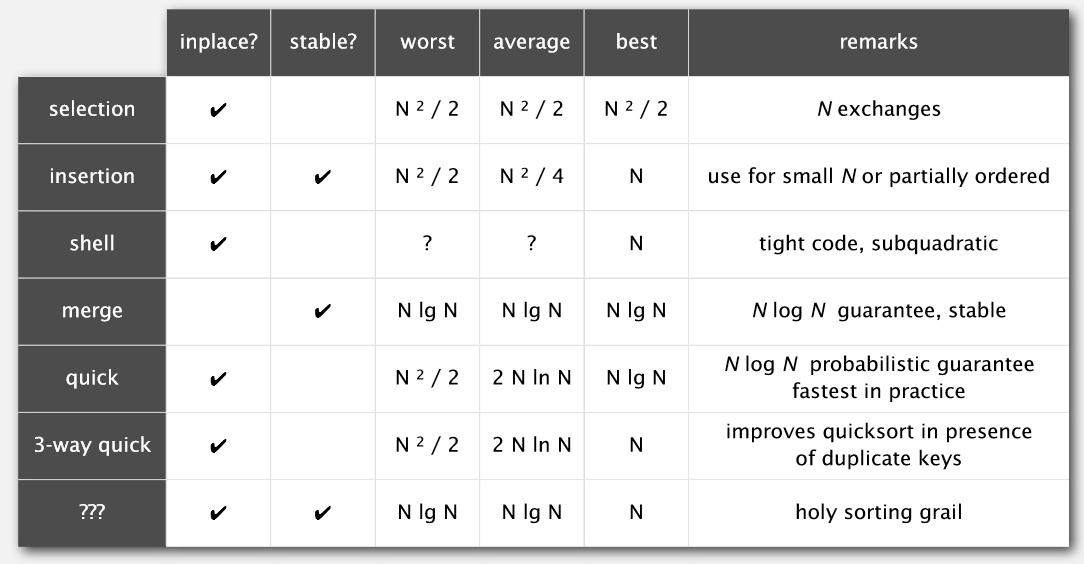应用

凸包

1. 选取二维平面上的点集中$y$轴坐标最小的点作为起始点p；
2. 将其他点按照它们与p之间的极角（polar angle）大小进行排序，极角相同的情况下比较与极点的距离，离极点比较近的优先；
3. 将p点与排序后的第一个点连接后，尝试依次将后面的点连接起来；
4. 如果连接某个点后，连接走向发生（如逆时针变成顺时针）变化，则抛弃之前连接的点，直到形成凸包。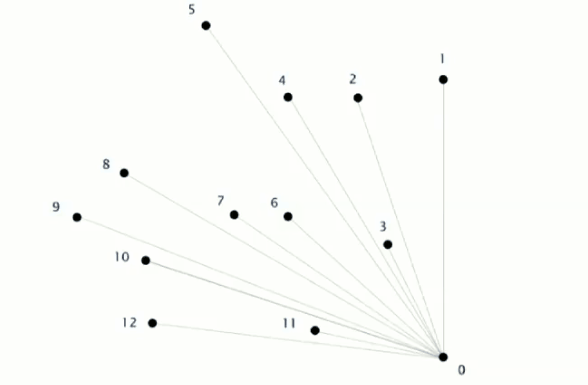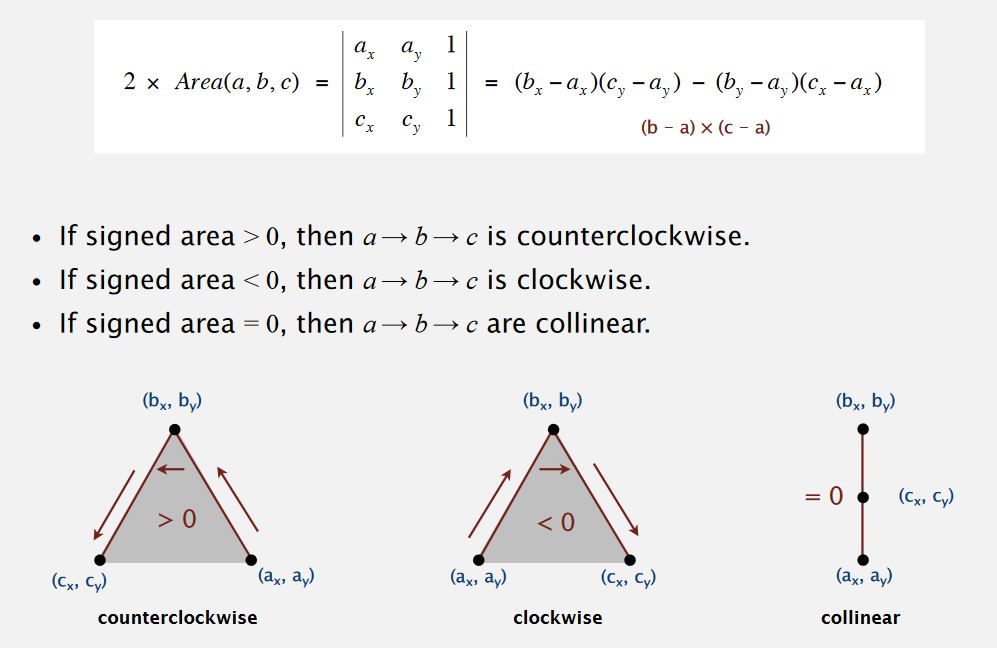参考资料

• 2019.03.31 完成初稿